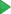# Testing Your First Python Application

In this section:

## Introduction

Remember, in the first tutorial you’ve created your first Python application, and in the second tutorial you’ve debugged it. Now it’s time to do some testing.

## Before you start

Open Settings/Preferences dialog (Ctrl+Alt+S), under the Tools node click Python Integrated Tools and select the default test runner - let it be `unittest` (refer to this page for details).

Note also that we are using unittest to test our application, but if you prefer to use another framework, PyCharm also supports nosetest and py.test.

## Creating test

Open the Python file `Solver.py` for editing (F4). In the class `Solver` right-click the editor background, point to Go To, and then choose Test (or just press Ctrl+Shift+T):

The pop-up appears, suggesting you to create a new test:

OK, let’s do it:

The new Python test class is created:

You see that there is the `import` statement, the test class, as required by the documentation, and the test method for the method `demo`, that just fails.

Run this test (Run ‘Solver_unittest’ on the context menu) and see it failing.

However, this failing behavior is by default - let’s now provide some meaningful contents, but before that some transformation is required.

## Transforming the source code

### Transforming the source code of the class Solver

First, let’s transform our code a little. Let’s add a check for the zero value of `d`, and place all input statements out of the class `Solver`:

```import math class Solver: def demo(self, a, b, c): d = b ** 2 - 4 * a * c if d > 0: disc = math.sqrt(d) root1 = (-b + disc) / (2 * a) root2 = (-b - disc) / (2 * a) return root1, root2 elif d == 0: return -b / (2 * a) else: return "This equation has no roots" if __name__ == '__main__': solver = Solver() while True: a = int(input("a: ")) b = int(input("b: ")) c = int(input("c: ")) result = solver.demo(a, b, c) print(result)```

Now you see the Run iconin the left gutter next to the block `if __name__ == '__main__':`. If you hover your mouse pointer over this icon, you will see a pop-up window describing the available commands:

Clicking this icon reveals the pop-up menu:

Choose Run ‘Solver’ and see your script running.

### Transforming the source code of the test class

We’ll add few methods that check the equation roots, and also the method setUp:

```import unittest from Solver import Solver class SolverTest(unittest.TestCase): def setUp(self): self.solver = Solver() def test_two_roots_1(self): self.assertEqual(self.solver.demo(2, 5, 3), (-1, -1.5)) def test_two_roots_2(self): self.assertEqual(self.solver.demo(2, -9, 7), (3.5, 1)) def test_one_root(self): self.assertEqual(self.solver.demo(2, 4, 2), -1) def test_no_roots(self): self.assertEqual(self.solver.demo(2, 1, 3), "This equation has no roots")```

Now run the test by right-clicking the editor background above the declaration of the class `SolverTest`. This time all the tests pass successfully: